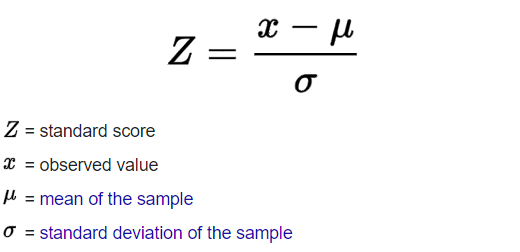# Z-score Method

Using Z-score method, we can find out how many standard deviations any particular value is away from the mean. The formula for Z-score is given as:If the Z-score of a data point is more than 3 (because it cover 99.7% of area), it indicates that the data value is quite different from the other values and so is considered as an outlier. Now let's use this method to detect outliers using Python.

INSTRUCTIONS
• First, let's import Numpy as `np`

``````import numpy as <<your code goes here>>
``````
• Now let's define an array of datapoints as `x` as follows

``````<<your code goes here>> = [5, 5, 5, -99, 5, 5, 5, 5, 5, 5, 88, 5, 5, 5]
``````
• Define a function `calculate_zscore` to find the outlier(s)

``````def <<your code goes here>>(data):
mean = np.mean(data)
std = np.std(data)
threshold = 2
outliers = []
for i in data:
z = (i-mean)/std
if abs(z) > threshold:
outliers.append(i)
print('outlier in dataset is', outliers)
``````
• Finally, let's call the function with our `x` set of datapoints to display the outliers

``````calculate_zscore(<<your code goes here>>)
``````

No hints are availble for this assesment

Answer is not availble for this assesment

Note - Having trouble with the assessment engine? Follow the steps listed here